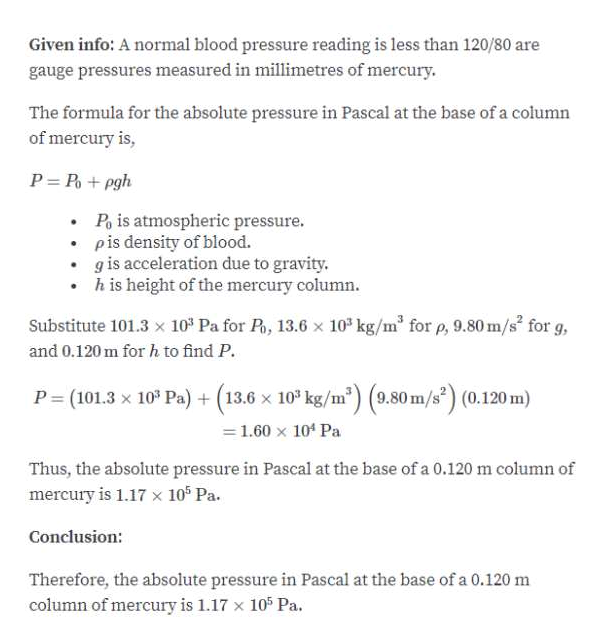# A normal blood pressure reading is less than 120/80 where both numbers are gauge pressures measured in millimeters of mercury (mmHg). What are the (a) absolute and (b) gauge pressures in pascals at the base of a 0.120 m column of mercury?

Question
76 views

A normal blood pressure reading is less than 120/80 where both numbers are gauge pressures measured in millimeters of mercury (mmHg). What are the (a) absolute and (b) gauge pressures in pascals at the base of a 0.120 m column of mercury?

check_circle

Step 1

Part A:...help_outlineImage TranscriptioncloseGiven info: A normal blood pressure reading is less than 120/80 are gauge pressures measured in millimetres of mercury. The formula for the absolute pressure in Pascal at the base of a column of mercury is, P= P + pgh Po is atmospheric pressure. pis density of blood. g is acceleration due to gravity. • h is height of the mercury column. Substitute 101.3 x 10° Pa for P, 13.6 x 10" kg/m for p, 9.80 m/s for g, and 0.120 m for h to find P. P = (101.3 x 10° Pa) + (13.6 x 10* kg/m) (9.80 m/s) (0.120 m) = 1.60 x 104 Pa Thus, the absolute pressure in Pascal at the base of a 0.120 m column of mercury is 1.17 x 10* Pa. Conclusion: Therefore, the absolute pressure in Pascal at the base of a 0.120 m column of mercury is 1.17 x 10 Pa. fullscreen

### Want to see the full answer?

See Solution

#### Want to see this answer and more?

Solutions are written by subject experts who are available 24/7. Questions are typically answered within 1 hour.*

See Solution
*Response times may vary by subject and question.
Tagged in

### Other